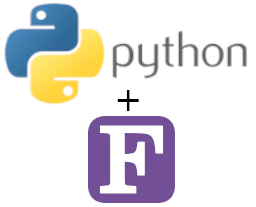# How to Call Fortran from Python

Combining the incredible flexibility of Python with Fortran for high-performance number-crunching is an excellent idea, especially if you already have some legacy Fortran code hanging around. Here's how to do it.Combine the numerical performance of Fortran with the wide scope and flexibility of Python

There are, esencially, two basic ways to call Fortran from Python:

• Use F2py library: this is the simplest and most established way, as the required wrappers are produced automatically. Regrettably, F2py supports a limited subset of Fortran.
• Combine iso_c_bindings on the Fortran side, with ctypes or Cython on the Python side. This is the most general way of using Fortran in Python.

For a thorough explanation of what is going on under the hood in the different alternatives, a good reference is the Numpy user manual section on ‘using Python as glue’, which you can find here.

In this tutorial, we will go through some quick examples. In particular, we will test the different methods of passing a complex Numpy array to Fortran.

## F2PY Example

This method is absolutely straightforward. Let’s say we have the following Fortran function,

!
!   my_fortran_lib.f90:
!
function foo(a) result(b)
implicit none

real(kind=8), intent(in)    :: a(:,:)
complex(kind=8)             :: b(size(a,1),size(a,2))

b = exp((0,1)*a)

end function foo

To compile it and create a Python extension module, use the following command: (pay attention to the “3” in F2py3 in case you intend to use Python 3).

f2py3 -c -m myflib my_fortran_lib.f90

That’s it, now the function is available to import it as a Python module. Simply call it by

import myflib
import numpy as np

a = np.array([[1,2,3,4], [5,6,7,8]], order='F')
myflib.foo(a)

Also, it is important to remember that C and Fortran employ different array orderings. Python normally employs the c-style row-major-order, while in Fortran the column-major-order is employed. By setting the Numpy order property to ‘F’ we are complying with the Fortran ordering.

## iso_c_bindings and ctypes example

The Fortran 2003 standard introduced iso_c_bindings as a means to improve interoperability with C. On the Python side, ctypes is a part of the stdlib which implements interfaces to foreign functions. These two tools can be combined to create an interface that enables Fortran and Python compatibility.

### 2.1 Getting started

As this is significantly more complicated than F2py we will start with a simple (yet meaningful) example, to get familiar with the basics. We will build a function that takes a double-precision float and adds the number 2.

As a first step, we need to declare the C types of each argument of our Fortran functions or subroutines. A good reference on how to translate each variable type can be found in the GCC docs here

In a file named my_fortran_lib.f90 we write:

function sum2(a) result(b) bind(c, name='sum2')
use iso_c_binding
implicit none

real(c_double), intent(in)  :: a
real(c_double)              :: b

b = a + 2.d0

end function sum2

We now compile this code as a shared library, with the command

gfortran -shared my_fortran_lib.f90 -o myflib.so

Now we have a shared library and we need to import it into Python. We use ctypes for this matter. It is also important to remember that, by default, Fortran passes values by reference.

import ctypes as ct

# import the shared library
fortlib = ct.CDLL('myflib.so')

# Specify arguments and result types
fortlib.sum2.argtypes = [ct.POINTER(ct.c_double)]
fortlib.sum2.restype = ct.c_double

# Create a double and pass it to Fotran (by reference)
a = ct.c_double(5)
b = fortlib.sum2(ct.byref(a))
print(b)

### 2.2 Dealing with complex numbers

Things start getting trickier here. Even though Fortran interoperability with C supports a ‘c_double_complex’ type which is part of the C99 standard, this is not supported by Python’s ctypes, at least at the time of writing this article. Follow this issue for updates on this matter. The workaround, for the moment, is to rely on wrapper functions, which can be easily coded in Fortran.

### 2.3 Dealing with multidimensional arrays

It is usually the case that we want to pass a large Numpy array to Fortran, as in our F2PY example, which also returns an array.

An important thing to notice is that the return type of a C-interoperable Fortran function can’t be an array. That’s because Fortran arrays are quite different from C arrays. The workaround is to rely on subroutines instead.

Our Fortran subroutine should look something like the following:

subroutine double_array(x,N) bind(C, name="double_array")
use iso_c_binding
implicit none

integer(c_int), intent(in), value   :: N
real(c_double), intent(inout)       :: x(N,N)

x  = exp(x)

end subroutine double_array

After compiling it as a shared library in the same way as before, we must build a Python interface. For this matter, we can resort to Numpy.ctypeslib to help us in building the required interface.

import ctypes as ct
import numpy as np

# import the shared library
fortlib = ct.CDLL('myflib.so')
f = fortlib.double_array

# Specify arguments type as a pointer to double and an integer
f.argtypes=[ct.POINTER(ct.c_double), ct.c_int]

# Create a double array, pass it to Fotran as a pointer
x = np.ones((3,3), order="F")
x_ptr = x.ctypes.data_as(ct.POINTER(ct.c_double))

# Call function
rint = f(x_ptr, ct.c_int(3))
print(x)